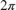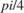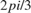# Write Script-Based Test Using Local Functions

This example shows how to write a script-based test that uses local functions as helper functions. The example function approximates the sine and cosine of an angle. The script-based test checks the approximation using local functions to check for equality within a tolerance.

### Create approxSinCos Function to Test

Create this function in a file, approxSinCos.m, in your current MATLAB folder. This function takes an angle in radians and approximates the sine and cosine of the angle using Taylor series.

function [sinA,cosA] = approxSinCos(x) % For a given angle in radians, approximate the sine and cosine of the angle % using Taylor series. sinA = x; cosA = 1; altSign = -1; for n = 3:2:26 sinA = sinA + altSign*(x^n)/factorial(n); cosA = cosA + altSign*(x^(n-1))/factorial(n-1); altSign = -altSign; end 

### Create Test Script

In your current MATLAB folder, create a new script, approxSinCosTest.m.

Note: Including functions in scripts requires MATLAB® R2016b or later.

%% Test 0rad % Test expected values of 0 [sinApprox,cosApprox] = approxSinCos(0); assertWithAbsTol(sinApprox,0) assertWithRelTol(cosApprox,1) %% Test 2pi % Test expected values of 2pi [sinApprox,cosApprox] = approxSinCos(2*pi); assertWithAbsTol(sinApprox,0) assertWithRelTol(cosApprox,1) %% Test pi over 4 equality % Test sine and cosine of pi/4 are equal [sinApprox,cosApprox] = approxSinCos(pi/4); assertWithRelTol(sinApprox,cosApprox,'sine and cosine should be equal') %% Test matches MATLAB fcn % Test values of 2pi/3 match MATLAB output for the sin and cos functions x = 2*pi/3; [sinApprox,cosApprox] = approxSinCos(x); assertWithRelTol(sinApprox,sin(x),'sin does not match') assertWithRelTol(cosApprox,cos(x),'cos does not match') function assertWithAbsTol(actVal,expVal,varargin) % Helper function to assert equality within an absolute tolerance. % Takes two values and an optional message and compares % them within an absolute tolerance of 1e-6. tol = 1e-6; tf = abs(actVal-expVal) <= tol; assert(tf, varargin{:}); end function assertWithRelTol(actVal,expVal,varargin) % Helper function to assert equality within a relative tolerance. % Takes two values and an optional message and compares % them within a relative tolerance of 0.1%. relTol = 0.001; tf = abs(expVal - actVal) <= relTol.*abs(expVal); assert(tf, varargin{:}); end 

Each unit test uses assert to check different output of the approxSinCos function. Typically, when you compare floating-point values, you specify a tolerance for the comparison. The local functions assertWithAbsTol and assertWithRelTol are helper functions to compute whether the actual and expected values are equal within the specified absolute or relative tolerance.

• Test 0rad tests whether the computed and expected values for an angle of 0 radians are within an absolute tolerance of 1e-6 or a relative tolerance 0.1%. Typically, you use absolute tolerance to compare values close to 0.

• Test 2pi tests whether the computed and expected values for an angle ofradians are equal within an absolute tolerance of 1e-6 or a relative tolerance 0.1%.

• Test pi over 4 equality tests whether the sine and cosine ofare equal within a relative tolerance of 0.1%.

• Test matches MATLAB fcn tests whether the computed sine and cosine ofare equal to the values from the sin and cos functions within a relative tolerance of 0.1%.

### Run Tests

Execute the runtests function to run the four tests in approxSinCosTest.m. The runtests function executes each test individually. If one test fails, MATLAB still runs the remaining tests. If you execute approxSinCosTest as a script instead of using runtests, MATLAB halts execution of the entire script if it encounters a failed assertion. Additionally, when you run tests using the runtests function, MATLAB provides informative test diagnostics.

results = runtests('approxSinCosTest'); 
Running approxSinCosTest .... Done approxSinCosTest __________ 

All the tests pass.

Create a table of test results.

rt = table(results) 
rt = 4x6 table Name Passed Failed Incomplete Duration Details _________________________________________ ______ ______ __________ ________ ____________ {'approxSinCosTest/Test0rad' } true false false 0.32861 {1x1 struct} {'approxSinCosTest/Test2pi' } true false false 0.024002 {1x1 struct} {'approxSinCosTest/TestPiOver4Equality' } true false false 0.030325 {1x1 struct} {'approxSinCosTest/TestMatchesMATLABFcn'} true false false 0.0439 {1x1 struct}